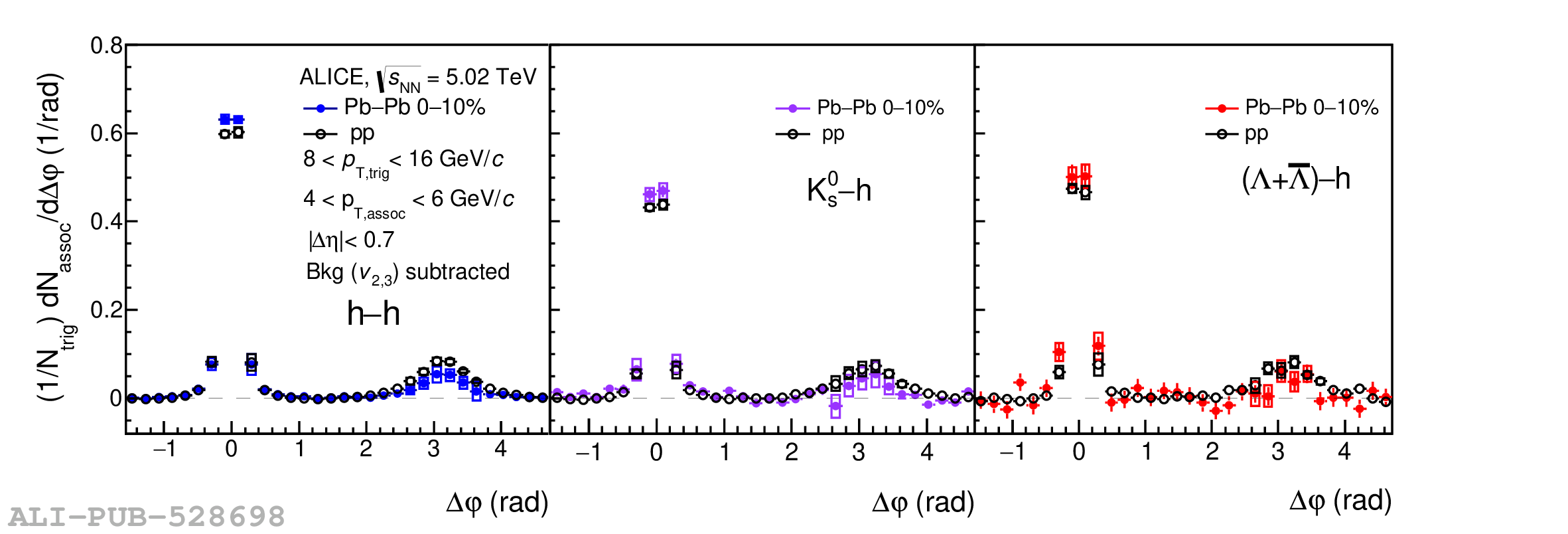# Figure 1

 Distributions of $C(\Delta\varphi)$ for h--h (left), \kzero--h (middle) and ($\Lambda$+\almb)--h (right) trigger particles with $8 < p_\mathrm{T,trig}<16$ \GeVc and associated particles with $4 < p_\mathrm{T,assoc}<6$ \GeVc in 0--10\% central \PbPb (solid points) and \pp (open circles) collisions The error bars represent the statistical uncertainties while the boxes represent the systematic uncertainties. The background has been subtracted based on the estimation of ZYAM in \pp collisions and the additional contributions of the anisotropic flow harmonics $v_{2}$ and $v_{3}$ in \PbPb collisions.